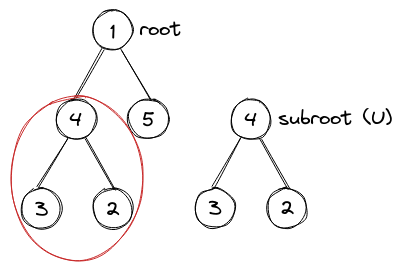# MangoSmashJONTRONARUET CodeSmash 2020
Limits 1.5s, 512 MB

Your friend has come to visit the RUET campus. As the Mango season goes on $\cdots$, he/she said “Mama Aam Khawa!”. As Manik Bhai leased those Mango Trees, he will not give you guys Mangoes for free rather he gave you a problem as he knew you are a great problem solver. You and your friend want one mango for each but with Distinction. The Distinction means bitwise $XOR$ of the sweetness of mangoes must be positive (greater than zero). There will be multiple queries.

Given a Mango Tree rooted at node $1$. Each node represents a Mango with sweetness $S_i$. Manik bhai will only let you choose mangoes from a subtree. So, in each query, Manik bhai will give you a node $U$ and you just need to find $-$ in the subtree rooted at $U$, how many ways you can take two mangoes such that they have Distinction.

A subtree of a tree is a tree that consists of a node in the tree and all of this node's descendants. The tree can also be considered as a subtree of itself.## Input

The first line of the input will have $N$ ($1 \leq N \leq 5 \times 10^5$) and $Q$ ($1 \leq Q \leq 5 \times 10^5$), number of Mangoes in the Tree and number of queries respectively.

Then the next line contains N numbers in form $S_i$ ($1 \leq S_i \leq {2^{31} -1}$), the sweetness of the $i$-th mango.

Next $N - 1$ lines, each containing $a$ ($1\leq a\leq N$) and $b$ ($1\leq b\leq N$) as there is a branch between node $a$ and $b$. It’s guaranteed that they will form a Tree.

The next line contains $Q$ numbers, indicating $U$, the root of the Subtree for each query.

## Output

For each query, print the answer of the Query. As the answer can be too large print modulo $1000000007$.

## Sample

InputOutput
5 5
1 2 2 3 2
1 2
2 4
1 3
2 5
1 2 3 4 5

7
2
0
0
0uDebug

### Submit